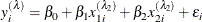The QLIM Procedure

MODEL Statement

Subsections:
• MODEL dependent = regressors < / options > ;

The MODEL statement specifies the dependent variable and independent regressor variables for the regression model.

You can specify the following options after a slash (/).

LIMIT1=ZERO |VARYING

specifies the restriction of the threshold value of the first category when the ordinal probit or logit model is estimated. LIMIT1=ZERO is the default option. When LIMIT1=VARYING is specified, the threshold value is estimated.

NOINT

suppresses the intercept parameter.

Endogenous Variable Options

The endogenous variable options are the same as the options that you can specify in the ENDOGENOUS statement. If you specify an ENDOGENOUS statement, all endogenous options in the MODEL statement are ignored.

Endogeneity and Overidentification Test Options

The endogeneity and overidentification test options are the same as the options that you can specify in the ENDOGENOUS statement. If you specify an ENDOGENOUS statement, all endogeneity and overidentification test options in the MODEL statement are ignored.

BOXCOX Estimation Options

BOXCOX (option-list )

specifies options that are used for Box-Cox regression or regressor transformation. For example, the Box-Cox regression is specified as

   model y = x1 x2 / boxcox(y=lambda,x1 x2)


PROC QLIM estimates the following Box-Cox regression model:The option-list takes the form variable-list < = varname > separated by commas. The variable-list specifies that the list of variables have the same Box-Cox transformation; varname specifies the name of this Box-Cox coefficient. If varname is not specified, the coefficient is called _Lambdai, where i increments sequentially.

Variable Selection Options

SELECTVAR <=(selectvar-option)>

enables variable selection. The selectvar-option specifies a variable selection method based on an information criterion. For more information, see the section Variable Selection. You can specify the following selectvar-options:

DIRECTION=FORWARD | BACKWARD

specifies the searching algorithm to use in the variable selection method. By default, DIRECTION=FORWARD.

CRITER=AIC | SBC

specifies the information criterion to use for the variable selection. By default, CRITER=AIC.

MAXSTEPS=value

specifies the maximum number of steps that are allowed in the search algorithm. The default is infinite; that is, the algorithm does not stop until the stopping criterion is satisfied.

LSTOP=value

specifies the stopping criterion. The value represents the percentage of decrease or increase in the AIC or SBC that is required for the algorithm to proceed; it must be a positive number less than 1. The default is 0.

RETAIN(regressors)

specifies a list of regressors that are to be retained in any model that the variable selection process considers.

The following rules apply to how regressors are handled when you specify more than one MODEL statement and use the SELECTVAR option:

• If you do not specify the SELECTVAR option in a particular MODEL statement, then all regressors in the original model are included in any model that the variable selection algorithm considers. In other words, omitting the SELECTVAR option is equivalent to providing the option: SELECTVAR=(RETAIN(all-regressors)).

• If you specify the SELECTVAR option without any =(option) clause in a MODEL statement, then all regressors in that model (other than the intercept, if present) are eligible for potential exclusion as the variable selection process is executed.

The following example specifies 10 possible regressor candidates, out of which five are selected using the AIC criterions:

   proc qlim data=one;
model y = x1-x10 /selectvar=(direction=forward criter=AIC maxsteps=5);
run;